Department of mathematics and physicsSmbat Shahinyan

Candidate of physical and mathematical Sciences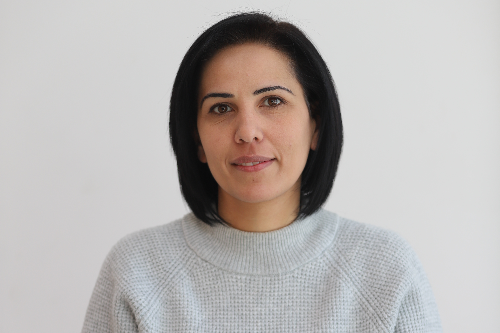Hermine Azizyan

Mathematics teacher

Candidate of physical and mathematical Sciences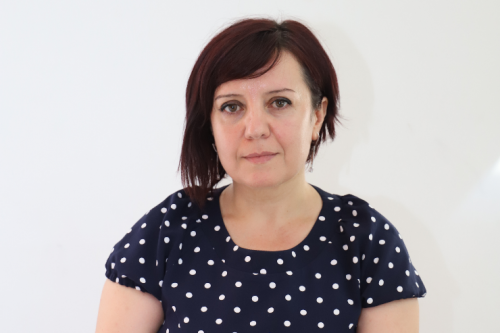Marine Broyan

Mathematics teacher

Candidate of physical and mathematical Sciences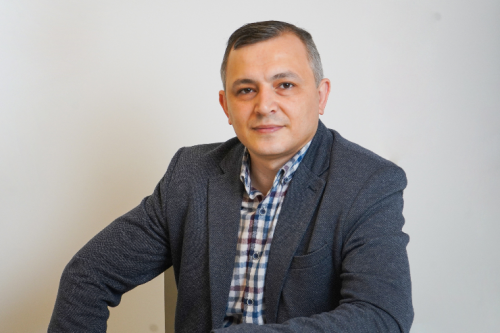Aram Matevosyan

Mathematics teacher

Candidate of physical and mathematical Sciences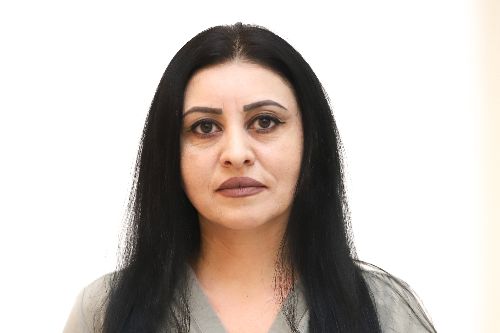Varsenik Nersisyan

Mathematics teacherYepraksya Israelyan

Mathematics teacherRuben Gevorgyan

Mathematics teacher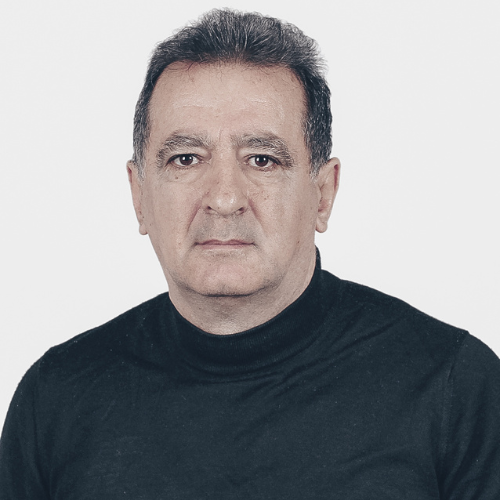Robert Zohranyan

Physics teacher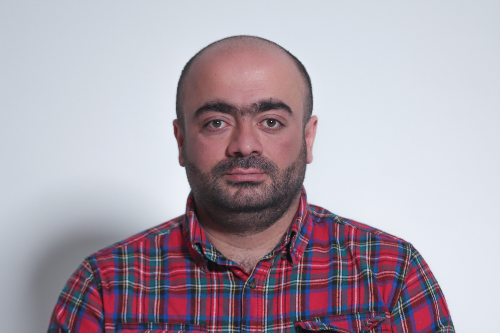Aghasi Mikaelyan

Physics teacher

Candidate of physical and mathematical SciencesLianna Mekhakyan

Laboratory assistant